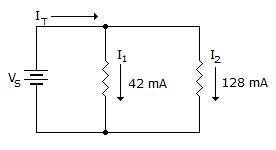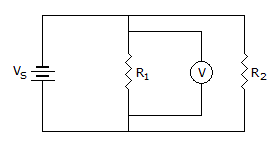# Electronics - Parallel Circuits

### Exercise :: Parallel Circuits - Filling the Blanks

11.If R1 opens in the given circuit, the total current equals ___.

 A. 86 mA B. 170 mA C. 42 mA D. 128 mA

Explanation:

No answer description available for this question. Let us discuss.

12.If R2 opens in the given circuit, the voltage across R1 will ___.

 A. increase B. decrease C. equal 0 V D. remain unchanged

Explanation:

No answer description available for this question. Let us discuss.

13.

A parallel resistor's value that changes with age will cause a corresponding change in ______ .

 A. branch current B. branch voltage C. both branch current and branch voltage D. power supply voltage

Explanation:

No answer description available for this question. Let us discuss.

14.

A circuit is parallel between two points if ___ between those two points.

 A. the voltage divides B. more than one current path exists C. all resistors are equal D. there is only one current path